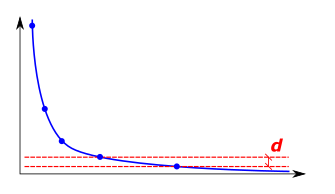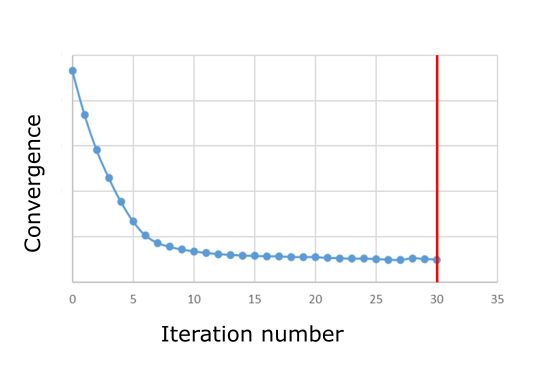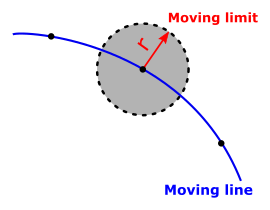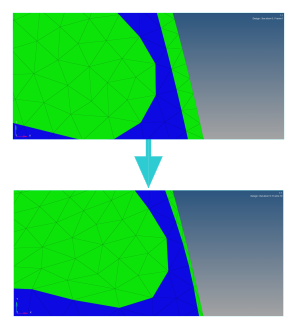# Optimization options

## Introduction

In the data tree of Flux the node Solver > Optimization > Optimization Options allows the user to set the different options for the optimization algorithm.

The table in the next section summarizes all the optimization options.

## Optimization options

Option Required informations
Optimization tolerance (in percent) Stops the optimization process when the objective value varies (d) less than the tolerance value between two iterations.Default value = 0.5%Maximum number of iterations Stops the optimization process when the maximum number of iterations is reached if the convergence was not met.Default value = 30Move limit Define an area where the nodes can move, this area may be defined by:
• A relative factor named r multiplied by the average element mesh size.Default value of the relative factor= 5.0or,
• An absolute move limit (in meter).Sensbilities filter Define an area where the nodes will move in an average direction composed of each node directions, this area may be defined as:
• A relative factor named r multiplied by the average element mesh size.Default value of the relative factor= 4.0or,
• An absolute radius (in meter).Tolerance for the barriers This tolerance is used to control how close the lines of the final design can be. 0.5 meaning half of a mesh size.Default value = 0.1Open a Flux with the final design when optimization is done
• Yes
• No